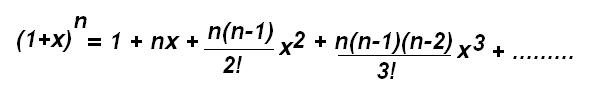# Help me in this simple prob..

## Recommended Posts

It doesn't hold. For example, let n = 10 and r = 3.

##### Share on other sites

No, but I can find plenty of counterexamples.

##### Share on other sites

sorry guys about some mistake ....but i am still confuse in this binomial expansion.....can u explain that part =>HOW IS C(n,2)x^2 = (n(n-1))/2!) OR C(n,3)x^3 = (n(n-1)(n-2))/(3!) of this equation....##### Share on other sites

See http://en.wikipedia.org/wiki/Binomial_theorem#Proof for a proof (by induction) of the binomial theorem. This appears only to prove the case where n is a positive integer. To be honest, I wouldn't mind seeing a proof of the general (real or complex) case myself.

##### Share on other sites

It just follows from the definition of nCr. For example, for nC3:

$\binom{n}{k} = \frac{n!}{k!(n-k)!} \Rightarrow \binom{n}{3} = \frac{n!}{3!(n-3)!}$

Now if we write this out, simply notice that most of the terms on the top and bottom cancel each other out:

$\binom{n}{3} = \frac{1}{3!} \cdot \frac{n \cdot(n-1) \cdot (n-2) \cdot (n-3) \cdot (n-4) \cdots 2 \cdot 1}{(n-3) \cdot (n-4) \cdots 2 \cdot 1} = \frac{n(n-1)(n-2)}{3!}$

##### Share on other sites

What do the !'s mean?

##### Share on other sites

TY Refsmmat :¬)

I understand

(n!)/(r!(n-r)!) = (n(n-1)) / 2!

To mean

n!/(r!*((n-r)!)) = (n*(n-1)) / 2

and that I could rearrange it like this

n!/(r!*((n-r)!)) - ((n*(n-1)) / 2) = 0

If n = 1 and r = 1

n!/(r!*((n-r)!)) = (n*(n-1)) / 2

1/0 = 0/2

inf = 2

If n = r

n!/(r!*((n-r)!)) = (n*(n-1)) / 2

n!/0 = (n*(n-1)) / 2

inf = (n*(n-1)) / 2

if n = 4 and r = 2

n!/(r!*((n-r)!)) = (n*(n-1)) / 2

(4*3*2)/(2*(((4*3*2)-2)!)) = ((4*3*2)*((4*3*2)-1)) / 2

24/(2*(22!)) = (24*23) / 2

<1 = 276

Have I got all my maths correctly and does this prove the equation incorrect?

Edited by alan2here
##### Share on other sites

0! is defined to be equal to 1, not 0. (Didn't check the rest, but I noticed that error straight off.)

##### Share on other sites

Thanks. Let me try again with the last part of my post.

If n = 1 and r = 1

n!/(r!*((n-r)!)) = (n*(n-1)) / 2

1 = 0

If n = r

n!/(r!*((n-r)!)) = (n*(n-1)) / 2

n!/(r!*1) = (n*(n-1)) / 2

if n = 4 and r = 2

n!/(r!*((n-r)!)) = (n*(n-1)) / 2

(4*3*2)/(2*2) = (4*3) / 2

24/4 = 12/2

6 = 6

So possibly

n!/(r!*((n-r)!)) = (n*(n-1)) / 2

Is true in some cases.

##### Share on other sites

nice question and nice answer by Alen

## Create an account

Register a new account